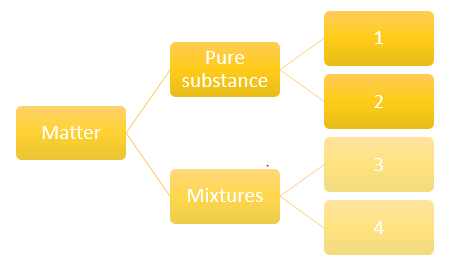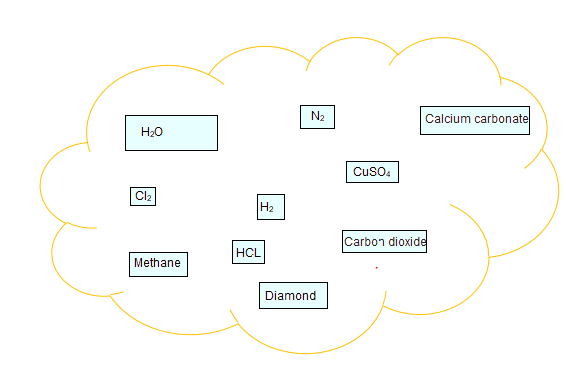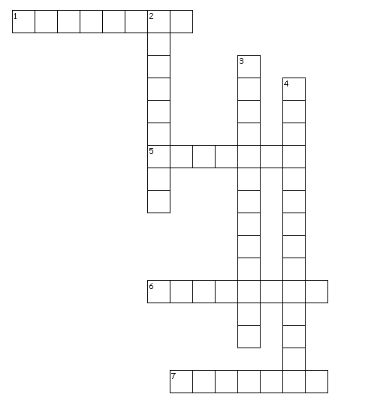# Extra Questions for Is Matter around us pure

Given below are the Class 9 Science Is matter around us pure extra questions with answers. These are important questions to prepare for examinationIt has below type to practice and excel in examination
(a) 1 Mark Questions
(b) Very Short questions
(c) Short questions
(d) Numericals Based on Concentration
(e) Long Anwer questions
(f) Crossword Puzzl
Hope you like them and do not forget to like , social share and comment at the end of the page.

## 1 Mark Questions

Question 1.
Salt can be recovered from its solution by evaporation. Can you suggest any other technique also?

Question 2.
Each Part Carry 1 Marks
Non-metals are usually poor conductors of heat and electricity. They are non-lustrous, non-sonorous, non-malleable and are coloured.
a.Name a lustrous non-metal.
b.Name a non-metal which exists as a liquid at room temperature.
c.The allotropic form of a non – metal is a good conductor of electricity. Name the allotrope.
d.Name a non-metal which is known to form the largest number of compounds.
e.Name a non-metal other than carbon which shows allotrophy.
f.Name a non-metal which is required for combustion.

Question 3.
Each Part Carry 1 Marks
Name the appropriate methods to separate the following:
a.Nitrogen from air
b.Dye from blue ink
c.Butter cream from milk
d.Ammonium chloride from common salt

Question 4.
Each Part Carry 1 Marks
Identify the physical and chemical changes from the following:
(i) Heating the mixture of iron and sulphur
(ii) Ripening of fruits
(iii) Dissolution of salt in water(iv) Rusting of iron – chair
(v) Making egg omelets

Question 5.
Each Part Carry 1 Marks
Classify the following into physical or chemical change:
(i) Burning of a candle
(ii) Freezing of water
(iii) Mixing of iron filings and sand (iv) Fading of clothes

Question 6.
Each Part Carry 1 Marks
(a) Name the separation technique you would follow to separate
(i) Dyes from black ink.
(ii) A mixture of salt and ammonium chloride
(iii) Cream from milk
(iv) Sodium chloride from its solution in water
(b) State the principle used in separating a mixture of two immiscible liquids.

Question 7. Each Part Carry 1 Marks
Give an example for each of following:
a)Solid -liquid homogeneous mixture
b)Gas- gas homogeneous mixture
c)Liquid -liquid heterogeneous misture

Question 1.
State any one difference between pure and impure substances.

Question 2.
What is meant by concentration of a solution?

Question 3.
List the two conditions essential for using distillation as a method for separation of the components from a mixture.

Question 4.
Smoke and fog both are aerosols. In what way are they different?

Question 5.
What do you understand by the statement 'the solubility of NaCl is 36.5 g at room temperature'?

Question 6
What is tincture of iodine? Identify the solute and solvent in it.

Question 7.
Can we separate alcohol dissolved in water by using a separating funnel? If yes, then describe the procedure. Of not, explain.

Question 8.
Crystallization is a better technique than sample evaporation. Give one reason to justify the statement.

Question 9.
Define an element.

Question 10.
What are metalloids? Give two examples.

Question 11.
Classify the following as metals, non-metals and metalloids:
Copper, silicon, carbon, chlorine, gold, boron.two non – metals from the following elements:

Question 12.
(a) Identify two non – metals from the following elements:
Carbon, Sodium, Chlorine, Neon, Platinum
(b) Name the appropriate method to separate nitrogen from air.
(c) Identify dispersed phase and dispersion medium in foam and rubber.

Question 13.
Identify the numbersQuestion 1.
How Tyndall effect can be observed in the canopy of a dense forest.

Question 2.
How do solultion and gel differ from each other? Give one example for each.
Question 3.
'Sea water can be classified as homogeneous as well as heterogeneous mixture.' Comment.
Question 4.
Explain why particles of a colloidal solution do not settle down when left undisturbed, while in the case of a suspension they do.
Question 5.
Mention in tabular form any two differences between heterogeneous and homogeneous mixtures.
Question 6.
What are aerosols? Give any two examples.
Question 7.
What is meant by an aqueous and non-aqueous solutions? Give one example of each.
Question 8.
What is a solution? Write two examples.
Question 9.
When is a solution said to be saturated? How can you change an unsaturated solution to a saturated solution without adding any more solvent to it?
Question 10.
Smoke and fog are aerosols. How do they differ from each other?
Question 11.
What is Tyndall effect? Why the solution of copper sulphate does not show Tyndall effect?

Question 12.
You are provided with solution of substance ‘X’. how will you test whether it is saturated or unsaturated with respect to ‘X’ at a given temperature? What happens when a hot saturated solution is allowed to cool?
Question 13.
What is chromatography? Mention its two applications.

Question 14.
Draw a flow diagram showing the process of obtaining gases from air.

Question 15.
State the separation technique used for the separation of the following:
a.Ammonium chloride from a mixture containing sodium chloride and ammonium chloride
b.Copper sulphate from its solution in water.

Question 16.
Classify the substances given in below figure into elements and compoundsQuestion 17.
Define solubility. How does solubility. How does solubility of a solid in water change with temperature?

Question 18.
Two students A and B were given 10 ml of water in a bowl and a plate respectively. They were told to observe the rate of evaporation. Name the student whose water evaporates faster and explain its reason.

Question 19.
Why the inter-conversion of states of matter is considered as a physical change? Give three reasons to justify your answer.
Question 20.
‘Colloidal solution appears to be homogeneous but actually it is heterogeneous.’ Give justification for this statement.
Question 21.
When a fine beam of light enters a room through a small hole, Tyndall effect is observed. Why does this happen? Give one more example where this effect can be observed.
Question 22.
With the help of a flow diagram, show the process of obtaining different gases from air. if the boiling point of oxygen, argon and nitrogen are 183° C, - 186° C and - 196° C respectively, which gas gets liquefied first as the air is cooled?

Question 23.
List three differences between a physical change and a chemical change.

Question 24.
Differentiate between, an element and a compound (any two points) with one example each.

Question 25.
(i) Name the compound formed on heating a mixture of iron filings and sulphur.
(ii) If dilute HCI is added to above compound then name the gas evolved and write down its two properties.

Question 26.
State any three differences between a mixture and a compound.

Question 27.
How will you separate a mixture of common salt, camphor and iron filings .Describe the process.

## Numericals

Question 1.
How many litres of 15% (mass/ volume) sugar solution would it take to get 75 g of sugar?

Question 2.
A solution contains 40 g of common salt in 320 g of water. Calculate the concentration in terms of mass by mass percentage of the solution.

Question 3.
A solution is prepared by adding 40 g of sugar in 100 g of water. Calculate the concentration in terms of mass by mass percentage of solution.

Question 4.
Calculate the mass of water and mass of glucose required to make 250 g of 40% solution of glucose.

Question 5.
During an experiment the students were asked to prepare a 10 % (Mass/ Mass) solution of sugar in water. Ramesh dissolved 10 g of sugar in 100 g of water while Sarika prepared it by dissolving 10 g of sugar in water to make 100 g of the solution.
(a)Are the two solutions of the same concentration?
(b)Compare the mass % of the two solutions.

Question 6.
Calculate the mass of sodium sulphate required to prepare its 20% (mass percent) solution in 100 g of water.

Question 7.
What volume of ethyl alcohol and water must be mixed together to prepare 250 ml of 60% by volume of alcohol in water.

Question 8.
A teacher told three students A, B and C to prepare 25% solution (mass by volume) of KOH. Student A dissolved 25 g of KOH in 100 g of water, student B dissolved 25 g of KOH in 100 mL of water and student C dissolved 25 g KOH in water and made the volume 100 mL. Which one of them has made required 25% solution? Give your answer with reason.

Question 9.
A solution contains 50 g of sugar in 350 g of water. Calculate the concentration of solution in terms of mass by mass percent of the solution.

Question 10.
To make a saturated solution, 72 g of sodium chloride is dissolved in 200 g of water at 200C. find its concentration at the same temperature.

## 5-Marks Questions

Question 1.
Write your observation when the following processes take place:
a. An aqueous solution of sugar is heated till it gets dried up.
b. A saturated solution of KCL at 60° C is allowed to cool at room temperature.
c. A mixture of iron filings and sulphur powder is heated strongly.
d. A bean of light is passed through colloidal solution.
e. Dilute HCI is added to mixture of iron filings and sulphur powder.

Question 2.
what is chromatography? How will you separate the components of black ink using chromatography? Write any two applications of chromatography.
Question 3.
(a) You are given a mixture of sand, water and mustard oil. How will you separate the components of this mixture? Explain it with the help of different separation methods involved in it.
(b) Give flow diagram showing the process of obtaining gases from air.
Question 4.
A.Which of the following are chemical changes and physical change?
(i) Decaying of wood
(ii) Sawing of wood
(iii) Burning of wood
(iv) Hammering of a nail into a piece of wood
B.We know that Tincture of iodine has antiseptic properties. This solution is made by dissolving
(a) iodine in alcohol
(b) iodine in vaseline
(c) iodine in $H_20$
(d) iodine in $KI$

Question 5.
To make a saturated solution 36 g of sodium chloride is dissolved in 100 g of water at 293 K. find its concentration at this temperature.

Question 6.
Give two examples from daily life where Tyndall effect is observed.
Question 7.
Identify homogeneous and heterogeneous mixtures among the following:
Air, salt solution, kerosene in water, muddy water, soil, soda water
Question 8.
Show diagrammatically how water is purified in the water works system and list the processes involved.

Question 9.
(i) What is meant by crystallization? How is impure copper sulphate purified by the process?
(ii) Differentiate between simple distillation and fractional distillation.

Question 10.
(a) Compare metals and non - metals based on their physical properties. (any four points)
(b) What are metalloids? Give two examples.
(c) Identify metals from the following: Boron, sodium, mercury, carbon.

Question 11.
Distinguish between homogeneous and heterogeneous mixtures. Classify the following mixtures as homogeneous and heterogeneous:
(i) Tincture of iodine (ii) Smaoke
(iii) Brass(iv) Sugar solution

## Crossword PuzzleAcross
1. hydrogen ______ is a color gas with a smell of rotten eggs
5. The major components in solution
6. Melting point and boiling point are _______ properties
7. Two elements are liquid at room temperature are mercury and _______
Down
2. In colliads ,The particles are called the ______ phase and the medium in which they are distributed is called the dispersion medium.
3. amount of solute present per unit volume or mass of the solution or solvent
4. denser particles are forced to the bottom and the lighter particles stay at the top when spun rapidly

## Summary

This Is matter around us pure extra questions with answers is prepared keeping in mind the latest syllabus of CBSE . This has been designed in a way to improve the academic performance of the students. If you find mistakes , please do provide the feedback on the mail.